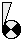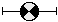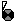# Parameters

The term 'parameter' usually refers to a symbol in an equation that can take on different values. When discussing equations in Simile, we use the term to describe the local names for values from other Simile components. However, here we are talking about quantities that are parameters for the whole model; special components whose values are specified externally and will change from one run to the next as the simulated conditions change. The values for these components come from outside the model; if they have equations, these are only used to set the dimensionality or provide default values.

There are two kinds of parameter that can be used in models. A variable in Simile can be marked as a fixed or variable parameter by ticking the appropriate radio button in the equation dialogue. A compartment can be a fixed parameter (i.e., its initial value is set externally) but not a variable parameter.Fixed parameter The value of a fixed parameter is set when the model is ready to run, and can be taken from a file, a table editor, or entered directly. It does not change its value during the simulation. In Simile v6 or lower, any value in the equation is ignored, and used only to set the parameter's dimensions. As of Simile v7, any value in the equation is used as a default if no other has been set.Variable parameter The value of a variable parameter is first set when the model is initialized or reset, and it can change throughout the simulation. Values can be taken from a file, a table editor, or using a slider control. The value in the equation is used as a default if no other has been set. If no value is entered, the default is the midpoint between the lower and upper bounds.

## Dimensionality

When you are adding file parameters to your model, it is important that they have the right dimensions for the data that they will hold. The file parameter dialogue only allows you to enter data that has the same dimensions as the parameter that is to represent it in the model. A fixed parameter that is not inside a multi-instance submodel will only accept a single numerical value. To enter an array of values, you must first make your parameter an array, either by putting it inside a fixed-membership multi-instance submodel, or by giving it an equation whose result has array dimensions, e.g., by using the makearray(...) function.

# Fixed parameters

A value of a fixed parameter is not stored with the model, but is supplied by the user when the model runs. The value cannot change during the simulation. To set the value of a fixed parameter use scenario files. These are loaded as the model is built or reset.

Use the "Units" field to define the units as integer (int), real or Boolean (Boolean). An equation can be entered whose dimensions will be used as the dimensions of the parameter data. In Simile v7 or later, the equation also sets its value if no value is entered via the parameter mechanism.

Use the equation dialogue box to designate a variable as a fixed parameter. It is also possible to set the initial value of a compartment in the same way. A fixed parameter is shown on the model diagram with apaper roll behind its usual appearance.

# Variable parameters

A value of a variable parameter is not stored with the model, but is supplied by the user when the model runs. The value can change during the simulation. There are several ways to set the value of a variable parameter. One is to use slider controls. These can be displayed in the run-time environment and provide a mechanism for the user to observe and adjust the value of the parameter. The other is to use scenario files. These are loaded as the model is built or reset, and can provide a time-series of values for the parameter.

Maximum and minimum values must be set to define the range of acceptable values for the parameter. This allows the slider control to be shown with the correct range. It is also possible to define whether the slider moves continuously or in integer increments. Use the "Units" field to define the units as integer (int) or real to effect this distinction. It is also possible to present a check-box to the user, to return the Boolean values true or false. This is accomplished by setting the "Units" field to boolean. Finally, a variable parameter can be an enumerated type; set the "Units" field to the name of the required type. In this case the slider helper will present a pull-down menu for the parameter, listing the type's members.

Use the equation dialogue box to designate a variable as an variable parameter. It is not possible to set the value of a compartment in the same way, as variable parameters get new values from outside during the execution of the model, while compartments' values are determined from their previous values by the rates of flows in and out. A variable parameter is shown on the model diagram with a slider bar behind its usual appearance.